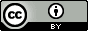## Monday, June 25, 2012

### Teaching computational chemistry with the ADF program

Célia Fonseca Guerra at the Vrije Universiteit Amsterdam has made the exercises she uses in teaching a bachelor level course on computational chemistry available here. The exercises are based on the ADF program, but could be adapted to other programs without too much trouble.

## Tuesday, June 5, 2012

### Complete basis set limit extrapolation

The complete basis set (CBS) limit is not a basis set though it is often written as such, e.g. B3LYP/CBS.  Instead the CBS limit is an extrapolated estimate of a result obtained using an infinitely large (complete) basis set.  In principle this procedure removes any error due to the linear combination of atomic orbitals approximation, and any remaining disagreement with experiment is due to some other approximation such as the treatment of correlation.  For many properties the CCSD(T)/CBS value can be regarded as a numerically exact for all practical purposes, i.e. it is unlikely that any higher level of theory predict significantly better results.

The extrapolation is based on a minimum of three separate calculations with increasingly larger basis sets.  CBS limit extrapolation works only with basis sets designed specifically for the task, such as the correlation- or polarization-consistent basis sets, e.g. cc-pVxZ or pc-n.

The procedure is as follows: a given property $Y$ of interest (e.g. a relative energy, a frequency, or a bond length) is computed at a given level of theory (e.g. B3LYP) using at least three basis sets (e.g. cc-VDZ, cc-VTZ, and cc-VQZ.  These data points a then fit to an equation, the two most popular equations are given here

$Y(x)=Y_{CBS}+Ae^{-Bx}$  (1)

$Y(x)=Y_{CBS}+Ax^{-3}$    (2)

Here, $Y_{CBS}$ is the CBS limit we're after and $x$ is 2 for cc-pVDZ, 3 for cc-pVTZ, and so on. $x$ is also often written as $L_{max}$ (or $l_{max}$), which is the highest angular momentum included in the basis set.  For cc-pVDZ this means $d$ orbitals, which have an angular momentum of 2, so $x$ and $L_{max}$ are really the same.

Equation (1) contains three parameters ($Y_{CBS}$, $A$, and $B$) so a minimum of three different basis sets are needed to determine them.  While Equation (2) only has two parameters, a minimum of three data points are still needed for reliable results.

For some properties and correlation methods the use of the double-zeta basis set does not lead to a good fit, so calculations with pentuple-zeta basis sets are necessary.   There is some evidence that the pc-n basis set provides faster convergence to the CMS limit.  CBS limit extrapolation is computationally very demanding and is typically done on relatively small systems to provide benchmark values to test more efficient methods.

Acknowledgment: I thank Anders Christensen for providing me with key papers and with helpful discussion.## Friday, June 1, 2012

### Computational Chemistry Highlights: May issue

The May issue of Computational Chemistry Highlights is out.

CCH is an overlay journal that identifies the most important papers in computational and theoretical chemistry published in the last 1-2 years.  CCH is not affiliated with any publisher: it is a free resource run by scientists for scientists.  You can read more about it here.

Table of content for this issue features contributions from CCH editors Steven Bachrach, Dean Tantillo, Dmitri Fedorov, and Jan Jensen:

On the aromatic stabilization of corannulene and coronene

ROBIA and Dolabriferol

Interested in more?  There are many ways to subscribe to CCH updates.